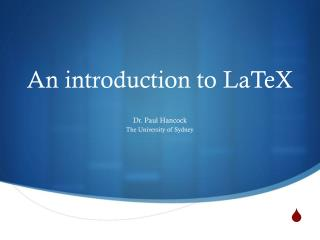DownloadDownload PresentationAn introduction to LaTeX

An introduction to LaTeX

Télécharger la présentationAn introduction to LaTeX

- - - - - - - - - - - - - - - - - - - - - - - - - - - E N D - - - - - - - - - - - - - - - - - - - - - - - - - - -
Presentation Transcript

1. An introduction to LaTeX Dr. Paul Hancock The University of Sydney

2. Overview • What is LaTeX? • Why use LaTeX? • How to use LaTeX • Common tricks • Further references/resources

3. What is LaTeX? • TeX is a typesetting system • LaTeX is a document markup language based on TeX • “a programming language that produces documents”

4. Why Use LaTeX? • LaTeX can typeset equations and symbols with ease • LaTeX can update citations and references automatically • LaTeX separates content and structure from formatting and style: • You provide content/structure (.tex files) • Publisher provides formatting and style (.sty files) • Ideally you spend more time on content than on formatting • Most (astronomy) journals require submissions to be in LaTeX

5. LaTeX example • You can access many symbols and characters without needing to choose crazy fonts that no-one else has • L_{Edd}=4\pi GMm_{p}c/\sigma_{T}\cong1.3\times10^{38}(M/M_{\odot})\,\mathrm{erg\: s^{-1}} becomes:

6. How to use LaTeX • Edit ms.tex in your favorite text editor (emacs/vim/etc) • “Compile” your document and open • latex ms.tex; xdvims.dvi or • pdflatexms.tex; acroreadms.pdf

7. latex or pdflatex? • “latex ms.tex” will produce .dvi files that can be read by xdvi • latex will leave spaces for eps/ps files which are then added in by xdvi when displaying your document • “pdflatexms.tex” will produce .pdf files that can be read by acrobat • “pdflatex” will embed png/jpg files within the pdf itself • .pdf files are more easily transported cross platform and are smaller in size than .dvi+eps files

8. Graphics format • You cannot use eps/ps and png/jpg graphics in the same document • Vector graphics • Resolution independent • Files size depends on content not image size • Can be manipulated within latex using psfrag • Raster graphics • Fixed resolution and file size • More easily portable (no fonts needed)

9. Basic components

10. Sections Use \section*{} for un-numbered sections

11. Figures • \begin{figure}\end{figure} Placement of figure (h! = here damnit!) Center figure within available space Caption text Size of figure Filename (extension optional) Label (must be *after* caption)

12. Tables Column alignment (left, right center) use | here for lines between columns \begin{tabular}makes columns & to separate columns Horizontal lines \\ to end row \caption must be after\end{tabular} Label (must be after caption)

13. Equations and symbols • Inline mathmode uses $...$ • Superscript uses ^, subscript uses _ • For more than a single character: use 10^{-15} or S_{radio}

14. Equations and symbols • Equation environment uses • \begin{equation} • \end{equation}

15. Large ([{| in equations • \left( \right) • \left{ \right} • \left| \right| • \left[ \right] • For matrices use \begin{array} \end{array} (cf. tabular) These will scale with the height of whatever they enclose, including nested brackets.

16. References and labels • Create labels with \label{fig:good_plot} \label{sec:introduction} • Reference with \ref{sec:introduction}

17. Citations and Bibliography • \usepackage{natbib} for easy bibliography • Create a bibliography file eg, ms.bib • Use \citep{Kormendy1995} when you want to cite the paper • See http://merkel.zoneo.net/Latex/natbib.php for more ways to cite papers/authors • At the end of your document write • \bibliographystyle{plainnat} • \bibliography{ms}

18. Creating a bibliography Type of entry (@article, @book, @inproceedings, …) Citation key for \citep{Kormendy1995} Bibliographic information

19. ADS can provide .bib entries Bibliographic entry that you can copy into your .bib file. Usually needs editing.

20. And then in the bibliography:

21. Getting bibtex to work • (pdf)latex/bibtex rely on each other so you must do: • Latex ms.tex % will complain about undefined \cite • Bibtexms % update ms.bbl and fix missing \cite • Latex ms.tex % replace ??? with actual citations • Latex ms.tex % fix long citations that overhang • Sometimes it all goes bad and you need to start fresh: • >rm *.aux *.bbl • \usepackage[utf8]{inputenc} to avoid problems with unicode. • eg, names like Königl

22. Citation management • JabREF – Java stand alone • Zotero – Browser plugin + stand alone • Mendeley – Online + stand alone • Papers2 –OSX/iOSstand alone • ADS native – online • Many more • No universal solution • Still better than by-hand management

23. Table of contents • \tableofcontents • Long section titles can be a problem • \section[short name]{long name} can avoid this • [short name] will appear in the ToC • {long name} appears in the text

24. List of figures/tables • \listoffigures • \listoftables • Captions will be used as labels. • \caption[short cap]{long cap} can avoid this • [short cap] appears in LoF/LoT • {long cap} appears in text

25. Typesetting Code • \usepackage{listings} % For highlighting code • \usepackage{color} % For colour • Provides syntax highlighting for many different languages • Import whole files with \lstinputlisting{example.py} • Short codes with • \begin{lstlisting}[language=matlab] • \end{lstlisting}

26. code.tex

27. Useful Resources • Examples and templates: • LaTeXthings.tar from • www.physics.usyd.edu.au/~hancock/index.php/Main/LaTeX • I will maintain this page and update with examples upon request • Send me your solutions to problems • Fellow students • PhD students and Postdocs are a good resource • (they have more time than professors)

28. Detexify • http://detexify.kirelabs.org/classify.html is an easy to use lookup service for latex commands